Home Data Analysis Black-Litterman Portfolio Allocation Model in Python

# Black-Litterman Portfolio Allocation Model in Python

A while ago I posted an article titled “INVESTMENT PORTFOLIO OPTIMISATION WITH PYTHON – REVISITED” which dealt with the process of calculating the optimal asset weightings for a portfolio according to the classic Markowitz “mean-variance” approach. With this method we aim to maximise our level of return for any given level of risk, in doing so we develop the concept of an “efficient frontier” and usually seek to identify the point/portfolio on that frontier which represents the best trade-off between risk and return (i.e. the portfolio with the highest Sharpe Ratio).

As nicely as the model allows us to identify our supposed “optimal asset weightings”, there are several, rather severe problems we face when using mean-variance optimisation.

1. The model assumes asset returns are normally distributed.
2. It can generate unintuitive, highly-concentrated portfolios.
3. The inputs to the model include each individual asset’s predicted/expected return and volatility, but we of course can never know those values for certain. Common practice is to calculate the assets’ historic returns and standard deviations and use them as proxies – this makes the massive presumption that all the assets will continue to behave and perform just as they have done in the past. Not only does it assume the returns and volatility will remain the same, it also assumes that correlations between all the assets in question will remain stable through time. We know these assumptions are just not realistic.
4. To make matters worse, not only do we use inputs that are reliant on our own “best guess” or forecasts, the model happens to be extremely sensitive to variations in these input values (especially to the return inputs, less so the volatility inputs). If the input values are changed, even by relatively small amounts, the optimal portfolio weightings created by the model can swing and vary wildly. Ideally we would like our model to be as “robust” as possible in this regard and generate stable/slowly changing suggested asset weightings when faced with changing input values.

In this post I am going to take a look at the Black-Litterman model, which is an adaptation of the classic mean-variance framework which enables investors to combine their unique views regarding the performance of various assets with the market equilibrium in a manner that results in intuitive, diversified portfolios.

The Black-Litterman model uses a Bayesian approach to combine the subjective views of an investor regarding the expected returns of one or more assets with the market equilibrium vector of expected
returns (the prior distribution) to form a new, mixed estimate of expected returns. The resulting new vector of returns (the posterior distribution), leads to intuitive portfolios with sensible portfolio weights.

This model combines the classic CAPM concept, reverse optimisation, mixed estimation, the “universal hedge ratio”/global CAPM and finally mean-variance optimisation, which we know is the foundation of the classic Markowitz framework discussed previously.

The problem of input-sensitivity outlined above, is overcome in the Black-Litterman model by the use of reverse-optimisation to back-out the required asset return vector (values) rather than them being an “exogenous” input as in the mean-variance model. We begin with the concept of “Equilibrium” returns as a neutral starting point – this set of “equilibrium” returns are the set of returns which are whatever is required so that the equilibrium asset allocation is equal to what we observe in the markets. That is to say, we can quite accurately calculate the market value of a certain set of assets or asset classes, and so their relative market values are used to extract what level of returns the market as a whole must be expecting in the future for those particular assets.

Imagine the whole global financial world as a huge investment portfolio managed by, in our case lets say God – if he had run a mean variance optimisation and then allocated the suggested amount of capital to each asset class, he would have ended up with a portfolio weighting that mimics what we actually see in the real world, right now.

The reason behind starting with equilibrium returns in this way is that it gives us a “sensible” set of predicted return values, which are based on solid economic foundations – later in the process the investor gets the chance to incorporate any subjective views or beliefs they may have into the input values by adjusting them accordingly.

Ok so how exactly do we calculate the equilibrium returns? They can be derived using a reverse optimization method in which the vector of implied excess equilibrium returns is extracted from known information using the following formula:

It’s worth noting here that the risk aversion parameter is set according to different approaches, depending on which practitioner you follow:

Some set their risk aversion parameter value to somewhere between 2.15 to 2.65 (as these values have the been the resulting recommendations of various research papers released on the subject)

Some set the value equal to the “market price of risk” (i.e. the risk aversion of the “Representative Investor”) which is computed as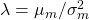(i.e. mean return of the global market portfolio divided by its variance). We shall use this method to set our risk aversion parameter value shortly.

Rearranging the above formula and substituting infor(withrepresenting any vector of excess return andrepresenting the vector of Implied Excess Equilibrium Returns) leads to the second formula shown below:

which is the solution to the unconstrained maximisation problem:

i.e. to makeequal to,has to be equal toNow I read in the csv data files containing historic return data for the universe of asset classes I am planning to include in my global portfolio, along with data regarding the market capitalisation based weights of each. The input files used can be downloaded using these following two links:

import numpy as np
import pandas as pd
from numpy.linalg import inv

cols = ['Global Bonds (Unhedged)','Total US Bond Market','US Large Cap Growth',
'US Large Cap Value','US Small Cap Growth','US Small Cap Value','Emerging Markets',
'Intl Developed ex-US Market','Short Term Treasury']
asset_returns = asset_returns_orig[cols].dropna()
treasury_rate = asset_returns['Short Term Treasury']
asset_returns = asset_returns[cols[:-1]].astype(np.float).dropna()
asset_weights = asset_weights.loc[cols[:-1]]

We can print out the average yearly returns and weights of each asset class as shown below:

asset_returns.mean()
asset_weights

Next we subtract the short term treasury rate from the asset class returns to obtain the relevant “excess returns” needed, generate the variance-covariance matrix of the excess returns. We then calculate the mean return and variance of the global market portfolio:

excess_asset_returns = asset_returns.subtract(treasury_rate, axis=0)
cov = excess_asset_returns.cov()
global_return = excess_asset_returns.mean().multiply(asset_weights['weight'].values).sum()
market_var = np.matmul(asset_weights.values.reshape(len(asset_weights)).T,
np.matmul(cov.values, asset_weights.values.reshape(len(asset_weights))))
print(f'The global market mean return is {global_return:.4f} and the variance is {market_var:.6}')
risk_aversion = global_return / market_var
print(f'The risk aversion parameter is {risk_aversion:.2f}')
The global market mean return is 0.0446 and the variance is 0.0202548
The risk aversion parameter is 2.20

Let’s write our first function which will help us reverse engineer the weights of a portfolio to obtain the Implied Equilibrium Return Vector.

def implied_rets(risk_aversion, sigma, w):

implied_rets = risk_aversion * sigma.dot(w).squeeze()

return implied_rets
implied_equilibrium_returns = implied_rets(risk_aversion, cov, asset_weights)
implied_equilibrium_returns

At this stage it is important that we familiarise ourselves with the “Black-Litterman Formula”. Throughout this article, K is used to represent the number of views and N is used to express the number of assets in the formula. The formula for the new Combined Return Vector is:

Very often, investment managers have specific views regarding the expected return of some of the assets in a portfolio, which differ from the Implied Equilibrium return. The Black-Litterman model allows such views to be expressed in either absolute or relative terms.

Let us set up 3 views we might have regarding some of the assets in our portfolio:

View 1: ‘Emerging Markets’ will have an absolute excess return of 9.25% (as opposed to the 7.62% equilibrium based value)

View 2: US Large Cap Growth and US Small Cap Growth will outperform US Large Cap Value and US Small Cap Value by 0.5% ((as opposed to the 1%-1.2% equilibrium based value)

View 3: ‘Intl Developed ex-US Market’ will have an absolute excess return of 5.5% (as opposed to the 6.31% equilibrium based value).

View 1 and 3 are examples of an absolute view, while view 2 is a relative view – FYI these relative type views tend to more closely represent the way most money/investment managers see the world and how they feel about different assets.

In the our example, the number of views () is 3; thus, the View Vector (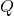) is a 3 x 1 column vector. The uncertainty of the views results in a random, unknown, independent, normally-distributed Error Term Vector () with a mean of 0 and covariance matrix. Thus, a view has the form.

The Error Term Vector () does not directly enter the Black-Litterman formula. However, the variance of each error term (), which is the absolute difference from the error term’s () expected value of 0, does enter the formula. The variances of the error terms () form, whereis a diagonal covariance matrix with 0’s in all of the off-diagonal positions. The off-diagonal elements ofare 0’s because the model assumes that the views are independent of one another. The variances of the error terms () represent the uncertainty of the views. The larger the variance of the error term (), the greater the uncertainty of the view.

Ourvector is as follows:

Q = np.array([0.0925, 0.005, 0.055])

We now set up Matrixwhich is where we input our views. As we have 3 views and 8 assets, we will create a 3 by 8 matrix as shown below:

P = [[0, 0, 0, 0, 0, 0, 1, 0],
[0, 0, .5, -.5, .5, -.5, 0, 0],
[0, 0, 0, 0, 0, 0, 0, 1]]

The first row of Matrix P represents View 1, where ‘Emerging Markets’ are the asset concerned – this is 7th along the top row to represent the fact it is the 7th asset class in our example (i.e. the 7th column in our original Pandas DataFrames (“asset_returns” and “asset_weights”)).

View 2 and View 3 are represented by Row 2 and Row 3, respectively.

The approach above can be considered an “equal weighting” approach which can sometimes cause relatively large changes in the proposed portfolio weightings of smaller asset classes. Some methods choose to weight the values in Matrix P by their market capitalisations, and this is the way we shall actually do it, so as to avoid any large swings in weightings.

From the asset class weights shown in our table, we can see the the Large Cap assets are near enough 8 times the size of the Small Cap assets. In our Matrix P we reflect this fact now as shown below:

P = np.asarray([[0, 0, 0, 0, 0, 0, 1, 0],
[0, 0, .85, -.85, .15, -.15, 0, 0],
[0, 0, 0, 0, 0, 0, 0, 1]])

The values in the middle row still sum to zero (as we are dealing with a relative, not absolute view) but now reflect the magnitude of their market capitalisation (0.9 vs 0.1 is 9 times larger, close enough to our 8 times value from our table).

It is now possible to calculate the variance of each individual portfolio view using the formula:, whereis a single 1 x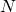row vector from Matrix P that corresponds to the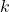th view andis the covariance matrix of excess returns.

view1_var = np.matmul(P.reshape(len(P)),np.matmul(cov.values, P.reshape(len(P)).T))
view2_var = np.matmul(P.reshape(len(P)),np.matmul(cov.values, P.reshape(len(P)).T))
view3_var = np.matmul(P.reshape(len(P)),np.matmul(cov.values, P.reshape(len(P)).T))
print(f'The Variance of View 1 Portfolio is {view1_var}, and the standard deviation is {np.sqrt(view1_var):.3f}\n',\
f'The Variance of View 2 Portfolio is {view2_var}, and the standard deviation is {np.sqrt(view2_var):.3f}\n',\
f'The Variance of View 3 Portfolio is {view3_var}, and the standard deviation is {np.sqrt(view3_var):.3f}')
The Variance of View 1 Portfolio is 0.09655215384615386, and the standard deviation is 0.311
The Variance of View 2 Portfolio is 0.014389680384615406, and the standard deviation is 0.120
The Variance of View 3 Portfolio is 0.04505784615384616, and the standard deviation is 0.212

This information is used shortly to revisit the variances of the error terms () that form the diagonal elements of.

There are several factors at play which influence the results of the Black-Litterman model, and it is conceptually a complex, weighted average of::

• Implied Equilibrium Return Vector ()
• the View Vector () (in which the relative weightings are a function of):
• the scalar ()
• the uncertainty of the views ()

The scalar () and the uncertainty of the views () are the most difficult model parameters to specify; the greater the level of certainty or confidence the manager expresses in the views, the closer the new return vector will be to the views. Vice versa, the less confidence the manager has regarding his view, the closer the new return vector will be to the original Implied Equilibrium Return Vector ().

The scalar tends to be more or less inversely proportional to the weight the model gives to the Implied Equilibrium Return Vector ().

The scalar tends to be set according to a number of different methods, depending on which well-known practitioner you choose to follow:

• Set the value of the scalar between 0.01 and 0.05, and then calibrate the model based on a target level of tracking error.
• Set the value of the scalar to 1.
• Set the value of the scalar to 1 divided by the number of observations.

For assets that are the subject of a view, the magnitude of their departure from their market capitalization weight is controlled by the ratio of the scalar () to the variance of the error term () of the view in question.

The easiest way to calibrate the Black-Litterman model is to make an assumption about the value of the scalar () (which we will set equal to 0.025) and then setting the ratio of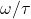equal to the variance of the view portfolio.

Using our value ofand the individual view variances calculated above, our covariance matrix of the error term () looks as follows (we create a function to generate our matrix):

def error_cov_matrix(sigma, tau, P):
matrix = np.diag(np.diag(P.dot(tau * cov).dot(P.T)))
return matrix
tau = 0.025
omega = error_cov_matrix(cov, tau, P)

Now let’s calculate our new “view based” return vector:

sigma_scaled = cov * tau
BL_return_vector = implied_equilibrium_returns + sigma_scaled.dot(P.T).dot(inv(P.dot(sigma_scaled).dot(P.T) + omega).dot(Q - P.dot(implied_equilibrium_returns)))

This gives us the below result:

BL_return_vector

Even though the expressed views only directly involved 6 of the 8 asset classes, the individual returns of all the assets changed from their respective Implied Equilibrium returns. We compare the new return vector with the original Implied Return Vector below:

returns_table = pd.concat([implied_equilibrium_returns, BL_return_vector], axis=1) * 100
returns_table.columns = ['Implied Returns', 'BL Return Vector']
returns_table['Difference'] = returns_table['BL Return Vector'] - returns_table['Implied Returns']
returns_table.style.format('{:,.2f}%')

We see that the “Emerging Markets” asset class return has risen by 0.29%, while the “Intl Developed ex-US Market” asset class return has fallen by 0.07%.

The relative changes between the “US Large Cap Growth” and the “US Large Cap Value” asset classes, and between the “US Small Cap Growth” and the “US Small Cap Value” asset classes are both in favour of the “Value” bases categories (i.e. Large Growth fell 0.29% while the Large Value only fell 0.07%, and the Small Growth fell 0.02% while the Small Value actually rose by 0.1%).

These changes make sense as our views incorporated an absolute increase in the return of the “Emerging Markets”, an absolute decrease in the return of the “Intl Developed ex-US Market” class and a relative increase in the performance of the 2 US Value classes vs the US Growth classes (the equilibrium returned a difference of around 1.2% in favour of the Growth classes, while our view had it at just 0.5% in their favour).

We can now calculate the new Black Litterman based weights vector as follows:

inverse_cov = pd.DataFrame(inv(cov.values), index=cov.columns, columns=cov.index)
BL_weights_vector = inverse_cov.dot(BL_return_vector)
BL_weights_vector = BL_weights_vector/sum(BL_weights_vector)

We compare the new weights vector with the original Market Cap Weights below and the Mean-Variance optimised weights (assuming we use the historic mean annual return as the return vector input):

# Calculate mean-variance optimised weights
MV_weights_vector = inverse_cov.dot(excess_asset_returns.mean())
MV_weights_vector = MV_weights_vector/sum(MV_weights_vector)
weights_table = pd.concat([BL_weights_vector, asset_weights, MV_weights_vector], axis=1) * 100
weights_table.columns = ['BL Weights', 'Market Cap Weights', 'Mean-Var Weights']
weights_table['BL/Mkt Cap Diff'] = weights_table['BL Weights'] - weights_table['Market Cap Weights']
weights_table.style.format('{:,.2f}%')

Not surprisingly we can see that”

1) The weights have fallen for the “US Large Cap Growth” (-6.24%) and “US Small Cap Growth” (-1.11%) classes, while they have risen for the “US Large Cap Value” (+6.66%) and “US Small Cap Value” classes (+1.17%).

2) The weights have risen for the “Emerging Markets” class (+6.42%).

3) The weights have fallen for the “Intl Developed ex-US Market” class (-7.17%).

and lastly:

4) The weights for the remaining two asset classes (“Global Bonds (Unhedged)” and “Total US Bond Market”) have remained practically static.

Below is a visualisation of the various asset class weightings corresponding to:

1) The Black-Litterman model (incorporating our 3 views).

2) The Market Capitalisation weightings.

3) The Mean-Variance model (using historic mean returns as inputs).

import matplotlib.pyplot as plt
N = BL_weights_vector.shape
fig, ax = plt.subplots(figsize=(15, 7))
ax.set_title('Black-Litterman Model Portfolio Weights Recommendation vs the Market Portfolio vs Mean-Variance Weights')
ax.plot(np.arange(N)+1, MV_weights_vector, '^', c='b', label='Mean-Variance)')
ax.plot(np.arange(N)+1, asset_weights, 'o', c='g', label='Market Portfolio)')
ax.plot(np.arange(N)+1, BL_weights_vector, '*', c='r',markersize=10, label='Black-Litterman')
ax.vlines(np.arange(N)+1, 0, BL_weights_vector, lw=1)
ax.vlines(np.arange(N)+1, 0, MV_weights_vector, lw=1)
ax.vlines(np.arange(N)+1, 0, asset_weights, lw=1)
ax.axhline(0, c='m')
ax.axhline(-1, c='m', ls='--')
ax.axhline(1, c='m', ls='--')
ax.set_xlabel('Assets')
ax.set_xlabel('Portfolio Weighting')
ax.xaxis.set_ticks(np.arange(1, N+1, 1))
ax.set_xticklabels(asset_weights.index.values)
plt.xticks(rotation=90, )
plt.legend(numpoints=1, fontsize=11)
plt.show()

One thing to notice is just how much the Mean-Variance weightings vary, with far more “extreme” allocations being seen (e.g. over 100% weighting in US Bonds and short positions in Global Bonds, US Large Cap Value and Intl Developed ex-US Market).

Before finishing I wanted to quickly rerun the model that we have just been through, but this time doing so using a pre-built 3rd party package called “mlfinlab”. My reasoning for this is firstly to run a quick validation of our results and attempt to verify their correctness by reconciling them with the results obtained using the “mlfinlab” module. Secondly I wanted to highlight and remind people of the fact that when dealing with these kind of classic financial concepts and accompanying models, most of the time there will already exist a number of well documented and well maintained 3rd party modules offering a range of related classes and functionality, all available for download and use through the “pip install” command.

The only reason I didnt use a pre-built package for the above analysis is that they can “hide” a lot of complexity behind a high-level API that can allow the creation and application of various models/classes/methods/functions in a line or two of code – I try to show things step by step in my posts as I believe that is of more use for people trying to learn.

Having said that, lets move onto using the “mlfinlab” to rerun the above analysis – I am not going to dive into to much detail here as I just want an output to reconcile my previous results against.

from mlfinlab.portfolio_optimization.bayesian import VanillaBlackLitterman
views = [0.0925, 0.005, 0.055]
pick_list = [
{"Emerging Markets": 1.0},

{"US Large Cap Growth": 0.85,
"US Large Cap Value": -0.85,
"US Small Cap Growth": 0.15,
"US Small Cap Value": -0.15},

{"Intl Developed ex-US Market": 1.0}]

bl = VanillaBlackLitterman()
bl.allocate(covariance=cov,
market_capitalised_weights=asset_weights,
investor_views=views,
pick_list=pick_list,
asset_names=cov.columns,
tau=tau,
risk_aversion=risk_aversion)

The Implied Equilibrium Return Vector generated by the model in this case is shown below – and we can see that the values are identical to the Implied Equilibrium Return Vector we generated previously:

bl.implied_equilibrium_returns.T

The Posterior Black-Litterman return vector generated by the model in this case is shown below – and we can see that the values are also identical to the Black-Litterman return vector we generated previously:

bl.posterior_expected_returns.T

The Black-Litterman recommended portfolio weights vector generated by the model in this case is shown below – in this case we can see that there are some small differences in the values compared to our previously calculated Black-Litterman recommended portfolio weights. I have included the earlier weights below for ease of comparison: the largest variation seen across all asset classes is just 0.4% (I believe the difference stems from the use of the original covariance matrix of excess returns when calculating the new weightings, rather than creating and using an updated “posterior” covariance matrix as they have in the mlfinlab model).

Long story short, I am satisfied that our earlier results have been corroborated as being correct.

weights_table2 = pd.concat([bl.weights.T, BL_weights_vector], axis=1) * 100
weights_table2.columns = ['mlfinlab', 'Initial Results']
weights_table2['Difference'] = weights_table2['Initial Results'] - weights_table2['mlfinlab']
weights_table2.style.format('{:,.2f}%')

Until next time…

#### You may also like

6 January 2021 - 08:59

i’m confused here…BL weights are extremely close to market cap weights while mean-variance optimized weights are totally not, so does that mean allocating asset by market cap is better than Markowitz optimal asset weightings?

6 February 2021 - 20:54

Hi there, I guess “better” is a subjective term but I think the main take-away from the results are that the BL is a way to overcome some of the weaknesses inherent in the simple mean-variance approach. The simple mean-variance model can result in the suggested optimal portfolio weights that are rather extreme in nature, or are poorly diversified portfolios that are heavily concentrated in a small sub-set of assets.

The second paragraph of the article lists the major flaws/weaknesses of the mean-variance approach – and the BL model is a way to overcome some of these by under-pinning the overall model and subsequent suggested optimal weightings with the idea that the current observable real-world asset weightings represent our starting point.

28 May 2021 - 18:24

Hi Sir,

Many thanks for your post. Please I have the same issue with black-litterman model. It’s the starting point.
How do you guys manage the Market Weights? I know the model. But when It comes to apply it in python, R or Excel by myself, It’s very difficult to obtain the market weights.

I have no issue with Q, P, Omega etc….only markets weights sucks

Ex: top US stock – from S&P500 (AMZN, FB, TSLA, JPM, JNJ).
portfolio Market Value MV=1*10^9 in USD

Market weights:
Wamzn = (Mkt Cap amzn)/ (total Mkt Cap SP500)?
Wfb = (Mkt Cap fb)/ (total Mkt Cap SP500)?
Wtsla = (Mkt Cap tsla)/ (total Mkt Cap SP500)?
….. and so

Leo

28 May 2021 - 20:21

Hi Leo, thanks for your comment. Really, the concept of the “market portfolio” is generally used as it encompasses, by definition, the whole market and offers investors the most diversified portfolio possible – i.e. all idiosyncratic risk is theoretically diversified away. This is considered often-times as the ‘best bang for your buck’ if you follow the notion that investors aren’t rewarded for holing idiosyncratic risk and that the “only free lunch” in finance is diversification.

So this leads me on to your situation; if you see you universe of possible investments for this exercise as being just those handful of stocks you listed, then collectively they are your “market portfolio” in essence. You would calculate the Market Capitalisation Weight of each stock as being the relative weight of each versus the combined market capitalisation of them all (e.g. AMZN, FB and TSLA have market caps of 100, 50, 50 then MV weights would be 50%, 25% and 25%).

The thing to keep in mind however is that when you are calculating the “excess returns” as a starting point to the covariance matrix and such, they must be calculated vs you unique benchmark – which is the subset of stocks you are talking about, weighted by market cap. So basically a market cap weighted index of those stocks in your universe.

29 May 2021 - 11:42

Hi S666
If i follow you, the total market cap = 200. therefore the obtained weights of 50%,25% and 25% are those that I have to use as Wmkt (market weights) when calculating Pi – the implied equilibrium returns?

Thanks
Leo

2 June 2021 - 13:12

Yes indeed

29 August 2021 - 05:17

Many thanks for the step by step tutorial, really well explained.
for the mean-variance optimization, is there a way to get positive weights without shorting ?

8 November 2021 - 00:32

Hello there,

Thanks for the great tutorial!

I also have a question regarding the initial market cap weights. I get how you would do it for individual stocks, but how did you determine the weights for the factor indexes, emerging markets, etc.
I am trying to implement the BL-model for several ETFs:

iShares Russell 2000 ETF (IWM) $iShares STOXX Europe 600 UCITS ETF conv. to$
iShares MSCI Emerging Markets ETF (EEM) $iShares Core Nikkei 225 ETF conv. to$
iShares 20+ Year Treasury Bond ETF (TLT) $iShares Core U.S. Aggregate Bond ETF (AGG)$

But I can’t figure out how to determine the market cap weights for the Implied Excess Equilibrium Vector.

Best Regards

Elias

15 January 2022 - 20:24

Hi

First, great articles and great blog – thank you.

Where did you get two CSV data from, so that we can update it in the future?
Also, would summing up all equities give a “global equities” index (or benchmark)?

Thanks

This website uses cookies to improve your experience. We'll assume you're ok with this, but you can opt-out if you wish. Accept Read More